# Inequality

A relation connecting two real numbers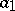and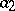by one of the symbols(less than),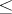(less than or equal to),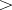(greater than),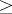(greater than or equal to),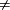(unequal to), that is,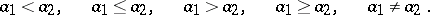Sometimes several inequalities are written together, for example,Inequalities have many properties in common with equalities. Thus, an inequality remains valid if one and the same number is added to (or subtracted from) both sides. Also, both sides can be multiplied by one and the same positive number. However, if both sides are multiplied by a negative number, the sign of an inequality is changed into the opposite (that is,is replaced byandby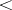). Fromand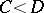it follows that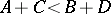and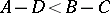, that is, inequalities with equal signs (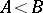and) can be added term-by-term, and inequalities with opposite signs (and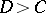) subtracted term-by-term. If,,, andare positive, thenandimplyand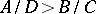, that is, inequalities with equal signs (between positive numbers) can be multiplied term-by-term and inequalities with opposite signs can be divided term-by-term.

Inequalities in which there occur quantities that can take distinct numerical values may be true for some such values and false for others. For example, the inequality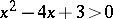is true for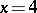and false for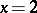. For inequalities of this kind the question arises of their solution, that is, of the bounds within which one must take the quantities occurring in the inequality so as to make it valid. Thus, by writingin the form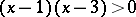one observes that it is true for allwith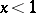or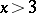, which are then the solutions of the given inequality.

Below some inequalities that hold identically in some range of variation of the variables occurring in them are given.

1) Inequality for the absolute value. For any real or complex numbers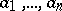: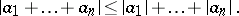2) Inequality for means. There are well-known inequalities connecting the harmonic, geometric, arithmetic, and quadratic mean (cf. Arithmetic mean; Geometric mean; Harmonic mean; Quadratic mean):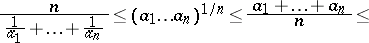Here all the numbersmust be positive.

3) Inequalities for sums and their integral analogues. Such are, for example, the Bunyakovskii inequality, the Hölder inequality, the Hilbert inequality, and the Cauchy inequality.

4) Inequalities for powers of numbers. Here the best known is the Minkowski inequality and its generalizations to the case of series and integrals.

5) Inequalities for certain classes of sequences and functions. Examples are the Chebyshev inequality for monotone sequences and the Jensen inequality for convex functions.

6) Inequalities for determinants. For example, Hadamard's inequality, see Hadamard theorem on determinants.

7) Linear inequalities. One considers a system of inequalities of the formThe set of solutions of this system of inequalities is a certain convex polyhedron in the-dimensional space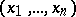; the task of the theory of linear inequalities (cf. Linear inequality) consists in the study of properties of this polyhedron.

Inequalities are of substantial value in all branches of mathematics. In number theory an entire section, Diophantine approximations, is completely based on inequalities; analytic number theory also operates frequently with inequalities (see, for example, Vinogradov estimates). In geometry, inequalities occur in the theory of convex bodies and in the isoperimetric problem (see Isoperimetric inequality; Isoperimetric inequality, classical). In probability theory many laws are stated by means of inequalities (see, for example, Chebyshev inequality in probability theory and its generalization, the Kolmogorov inequality). In the theory of differential equations one uses so-called differential inequalities (cf. Differential inequality). In function theory and approximation theory one uses various inequalities for derivatives of polynomials and trigonometric polynomials (see, for example, Bernstein inequality, Jackson inequality); on inequalities connected with the imbedding of classes of differentiable functions, see Kolmogorov inequality; Imbedding theorems. In functional analysis, in the definition of the norm in a function space, one requires the norm to satisfy the triangle inequality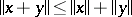. Many classical inequalities determine in essence the value of the norm of a linear functional or linear operator in one space or another or give estimates for them (see, for example, Bessel inequality; Minkowski inequality). In computational mathematics inequalities are used to estimate errors in the approximate solution of a problem.